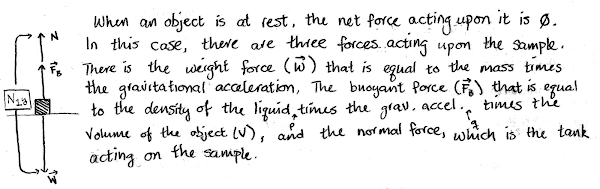## 20150105

### Physics final exam question: submerged metal sample resting at bottom

Physics 205A Final Exam, fall semester 2014
Cuesta College, San Luis Obispo, CA

Cf. Giambattista/Richardson/Richardson, Physics, 2/e, Problems 9.29(a), 9.31

A physics question on an online discussion board[*] was asked:
You're told the volume of a metal sample that is sitting fully submerged at the bottom of a tank filled with water. You're given the density of the water and the density of the metal. Why is the buoyant force equal to ρ·g·V, but not equal to the weight of the sample?
Discuss (a) why the buoyant force of the water on the sample is equal to ρ·g·V (specify which substances ρ and V refer to), and (b) why the buoyant force is not equal to the weight of the sample. Explain your reasoning using the properties of densities, volumes, forces, Newton's laws, Archimedes' principle (buoyant forces), and a free-body diagram.

• p:
Correct. Discusses:
1. that the density ρ and the volume V in the buoyant force equation refer to the density of water and of the entire sample, respectively;
2. the buoyant force is not equal to the weight of the sample, as the weight of the sample equals the two upwards forces on the sample: the buoyant force and the normal force, from discussion of Newton's first law and/or shown on a free-body diagram.
• r:
Nearly correct, but includes minor math errors. One of the two points (1)-(2) correct, other is problematic/incomplete.
• t:
Nearly correct, but approach has conceptual errors, and/or major/compounded math errors. Only one of the two points (1)-(2) correct, other is missing, or both are problematic.
• v:
Implementation of right ideas, but in an inconsistent, incomplete, or unorganized manner. Some garbled attempt at applying Archimedes' principle and Newton's laws.
• x:
Implementation of ideas, but credit given for effort rather than merit.
• y:
Irrelevant discussion/effectively blank.
• z:
Blank.
Sections 70854, 70855, 73320
Exam code: finalm34T
p: 15 students
r: 14 students
t: 19 students
v: 10 students
x: 2 students
y: 1 student
z: 1 student

A sample "p" response (from student 9950):